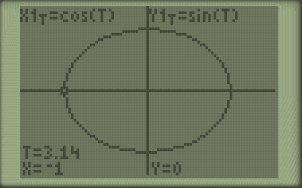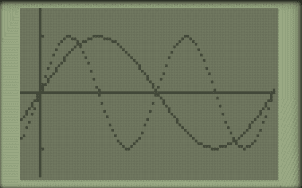# Period of Trig Functions

In this post, we’ll talk about the B term. This term helps us find the period, the distance required to complete one cycle. It multiplies the angle. For example y=sin(90°) or in radians, y=sin (π/2) is 1.y=sin(pi/2)=1

But if you make B=2 instead of 1 (not shown but assumed in the previous equation), it now looks like y=sin(2(90°) which simplifies to y=sin(2(180°). Or in radians, y=sin(2(π/2)) which simplifies to y=sin(π). These now equal 0.y=sin(pi)=0

To find the period, note that period=2π/B. So in this second example, 2π/2=π. In the first example, 2π/1=2π. In other words, the second example completes a complete cycle in only π radians (180°), whereas it takes 2π in the first example.dashed line is y=sin(x), solid is y=sin(2(x))key